Author Message
LiquidCode
Moderator Team

Joined: 05 Dec 2000
Posts: 1731
Location: Space and TimePosted: Thu Feb 11, 2010 11:37 pm    Post subject: Random Name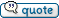This code creates a random string of letters. Good for creating random file names.

Useage: @RandomName(<Number of chars>)
Default is 4 id <Number of Chars> is left blank.

 Code: #Define function,RandomName :RandomName   #%1 = num of chars, blank for default of 4   %c = "abcdefghijklmnopqrstuvwxyz"   if @not(%1)      %1 = 4   end   RANDOM @datetime(shn)     %i = 1     repeat       %p = %p@substr(%c,@random(1,@len(%c)))       %i = @succ(%i)     until @equal(%i,@succ(%1))   exit @upper(%p)

_________________
Chris
Http://vdsfreak.com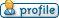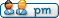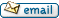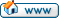uvedese
Contributor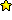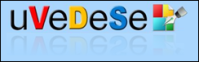Joined: 21 Jan 2006
Posts: 159
Location: SpainPosted: Fri Feb 12, 2010 4:43 pm    Post subject:Hi LiquidCode:

Very useful code. It can add an formatting argument, this way:

 Code: #Define function,RandomName :RandomName   #%1 = num of chars, blank for default of 4   #%2 = format string where N=number and L=letter; letter for default   %c = "abcdefghijklmnopqrstuvwxyz"   if @not(%1)      %1 = 4   end   RANDOM @datetime(shn)   %i = 1   if @greater(%1,@len(%2))     %2 = %2@fill(@diff(%1,@len(%2)),L)   end       repeat     if @equal(@substr(%2,%i),N)       %p = %p@random(0,9)     else       %p = %p@substr(%c,@random(1,@len(%c)))     end       %i = @succ(%i)   until @equal(%i,@succ(%1)) exit @upper(%p)

We can define the format of the string:

 Code: %a = @randomname(8,NNLLLLLL)

___________

uVeDeSe
___________SnarlingSheep
Professional Member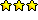Joined: 13 Mar 2001
Posts: 759
Location: MichiganPosted: Fri Feb 12, 2010 5:53 pm    Post subject:Option for either <Length> or <Format String> and added characters:
*Edited to remove invalid filename characters.
 Code: #Define function,RandomName info @RandomName(6) info @RandomName(NNLLNNCCC) exit :RandomName   #%1 = num of chars, blank for default of 4 or format string where N=number and L=letter; letter for default   %c = "abcdefghijklmnopqrstuvwxyz"   %d = "~!@#\$%^&()_+`-={}[];',."   if @not(%1)      %1 = 4   end   RANDOM @datetime(shn)   %i = 1   if @not(@numeric(%1))     %2 = %1     %1 = @len(%1)   end       repeat     if @equal(@substr(%2,%i),N)       %p = %p@random(0,9)     else       if @equal(@substr(%2,%i),C)         %p = %p@substr(%d,@random(1,@len(%d)))       else         %p = %p@substr(%c,@random(1,@len(%c)))       end     end

_________________
-Sheep
My pockets hurt...

Last edited by SnarlingSheep on Sat Feb 13, 2010 2:20 pm; edited 1 time in totaluvedese
ContributorJoined: 21 Jan 2006
Posts: 159
Location: SpainPosted: Fri Feb 12, 2010 6:43 pm    Post subject:If it wants to generate a consistent string as a valid file name, it must eliminate some characters:

 Code: %d = "~!@#\$%^&()_+`-={}[];',."

___________

uVeDeSe
___________LiquidCode
Moderator Team

Joined: 05 Dec 2000
Posts: 1731
Location: Space and TimePosted: Tue Apr 26, 2011 4:11 pm    Post subject:I revisited this code. I needed to generate many random names in more than a second and since the seed is based on the time it only changes every second. So I take it farther and also add a Random_Seed variable that is generated from the previous seed. So in theory, no two names should be the same when generated in less than a second.

 Code: #Define function,RandomName #info @RandomName(6) list create,1 %x = 0 repeat      list add,1,@RandomName(NNLLNN)      %x = @succ(%x) until @equal(%x,10) info @text(1) exit :RandomName   #%1 = num of chars, blank for default of 4 or format string where N=number and L=letter; letter for default   %c = "abcdefghijklmnopqrstuvwxyz"   %d = "~!@#\$%^&()_+`-={}[];',."   if @not(%1)      %1 = 4   end   if %%Random_seed      Random %%Random_Seed   else      RANDOM @datetime(shn)@random(0,9)   end   %i = 1   if @not(@numeric(%1))     %2 = %1     %1 = @len(%1)   end   repeat     if @equal(@substr(%2,%i),N)       %p = %p@random(0,9)     else       if @equal(@substr(%2,%i),C)         %p = %p@substr(%d,@random(1,@len(%d)))       else         %p = %p@substr(%c,@random(1,@len(%c)))       end     end     %i = @succ(%i)   until @equal(%i,%1)   %%Random_Seed = @random(1000)  exit @upper(%p)

_________________
Chris
Http://vdsfreak.comDisplay posts from previous: All Posts1 Day7 Days2 Weeks1 Month3 Months6 Months1 Year Oldest FirstNewest First
 All times are GMT Page 1 of 1